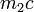# Isoclinic groups have same proportions of conjugacy class sizes

## Statement

Suppose$G_1$ and$G_2$ are finite groups that are isoclinic groups. Suppose$c$ is a positive integer. Denote by$m_1$ the number of conjugacy classes of$G_1$ of size$c$ and denote by$m_2$ the number of conjugacy classes of$G_2$ of size$c$. Then,$m_1$ is nonzero if and only if$m_2$ is nonzero, and if so, we have:$\frac{m_1}{m_2} = \frac{|G_1|}{|G_2|}$

In other words, given the conjugacy class size statistics of$G_1$, we can obtain the conjugacy class size statistics of$G_2$ by scaling the number of occurrences of each conjugacy class size by a factor of$|G_2|/|G_1|$.

In particular, if$G_1$ and$G_2$ also have the same order, then they have precisely the same conjugacy class size statistics.

## Relation with Schur covering groups

All the Schur covering groups of a given finite group are isoclinic groups, hence have the same conjugacy class size statistics.

## Facts used

1. Size of conjugacy class equals index of centralizer

## Proof

### Proof outline

The idea behind the proof is to show that the size of the conjugacy class of an element depends only on its coset modulo the center, and is completely determined by the information of the commutator map. We use Fact (1).

### Proof details

This proof uses a tabular format for presentation. Provide feedback on tabular proof formats in a survey (opens in new window/tab) | Learn more about tabular proof formats|View all pages on facts with proofs in tabular format

Given: Two isoclinic groups$G_1$ and$G_2$, a positive integer$c$.$m_1$ and$m_2$ are respectively the number of conjugacy classes in$G_1$ and$G_2$ of size$c$. Note that the actual number of elements in$G_1$ and$G_2$ with these conjugacy class sizes are$m_1c$ and$m_2c$ respectively.

To prove:$m_1$ is nonzero if and only if$m_2$ is nonzero, and if so,$\frac{m_1}{m_2} = \frac{|G_1|}{|G_2|}$

Proof: Let$W$ be the group$\operatorname{Inn}(G_1) \cong \operatorname{Inn}(G_2)$ and$T$ be the group$G_1' \cong G_2'$. Denote by$\gamma: W \times W \to T$ the map obtained from the commutator map in either group (we know both maps are equivalent via the isoclinism). Denote by$\alpha_1:G_1 \to W$ and$\alpha_2:G_2 \to W$ the quotient maps modulo the respective centers.

Step no. Asssertion/construction Facts used Given data used (column to be filled) Previous steps used Explanation
1 For$w \in W$, the centralizer in$G_1$ of any element in$\alpha_1^{-1}(w)$ is precisely$\alpha_1^{-1}(\mathcal{C}(w))$ where$\mathcal{C}(w) = \{ u \in W \mid \gamma(u,w) \mbox{ is the identity element of } T \}$. This follows by definition of centralizer.
2 For any$w \in W$, the size of the conjugacy class in$G_1$ of any element in$\alpha_1^{-1}(w)$ is the index of the subgroup$\mathcal{C}(w) = \{ u \in W \mid \gamma(u,w) \mbox{ is the identity element of } T \}$ in$W$. Fact (1) Step (1) By Fact (1), the conjugacy class size is$[G_1:\alpha_1^{-1}(\mathcal{C}(w))]$, which, by the fourth isomorphism theorem, is$[W:\mathcal{C}(w)]$.
3 The set of elements of$G_1$ with conjugacy class size$c$ is$\alpha_1^{-1}(S)$ where$S$ is the set of$w \in W$ for which the index of the subgroup$\mathcal{C}(w) = \{ u \in W \mid \gamma(u,w) \mbox{ is the identity element of } T \}$ in$W$ is$c$. Step (2)
4$m_1c = |S||Z(G_1)|$ where$S$ is as defined in Step (3). Both sides of the equality represent the number of elements in conjugacy classes of size$c$ in$G_1$. Step (3) follows directly, since the set is the union of$|S|$ many cosets, each of size$|Z(G_1)|$.
5$m_2c = |S||Z(G_2)|$ where$S$ is as defined in Step (3). Both sides of the equality represent the number of elements in conjugacy classes of size$c$ in$G_2$. Analogous to reasoning for Steps (1)-(4) Replace$G_1$ and$\alpha_1$ with$G_2$ and$\alpha_2$ throughout. Note that$S$ is defined in the same way in both cases.
6$m_1$ is nonzero if and only if$m_2$ is nonzero, and if so,$m_1/m_2 = |Z(G_1)|/|Z(G_2)|$. Steps (4), (5) Direct by taking the quotient of the descriptions.
7$m_1$ is nonzero if and only if$m_2$ is nonzero, and if so,$m_1/m_2 = |G_1|/|G_2|$. Step (6) We have that$[G_1:Z(G_1)] = [G_2:Z(G_2)]$ since the inner automorphism groups are isomorphic, so rearranging gives$|G_1|/|G_2| = |Z(G_1)|/|Z(G_2)|$. Combine with Step (5) to get the result.